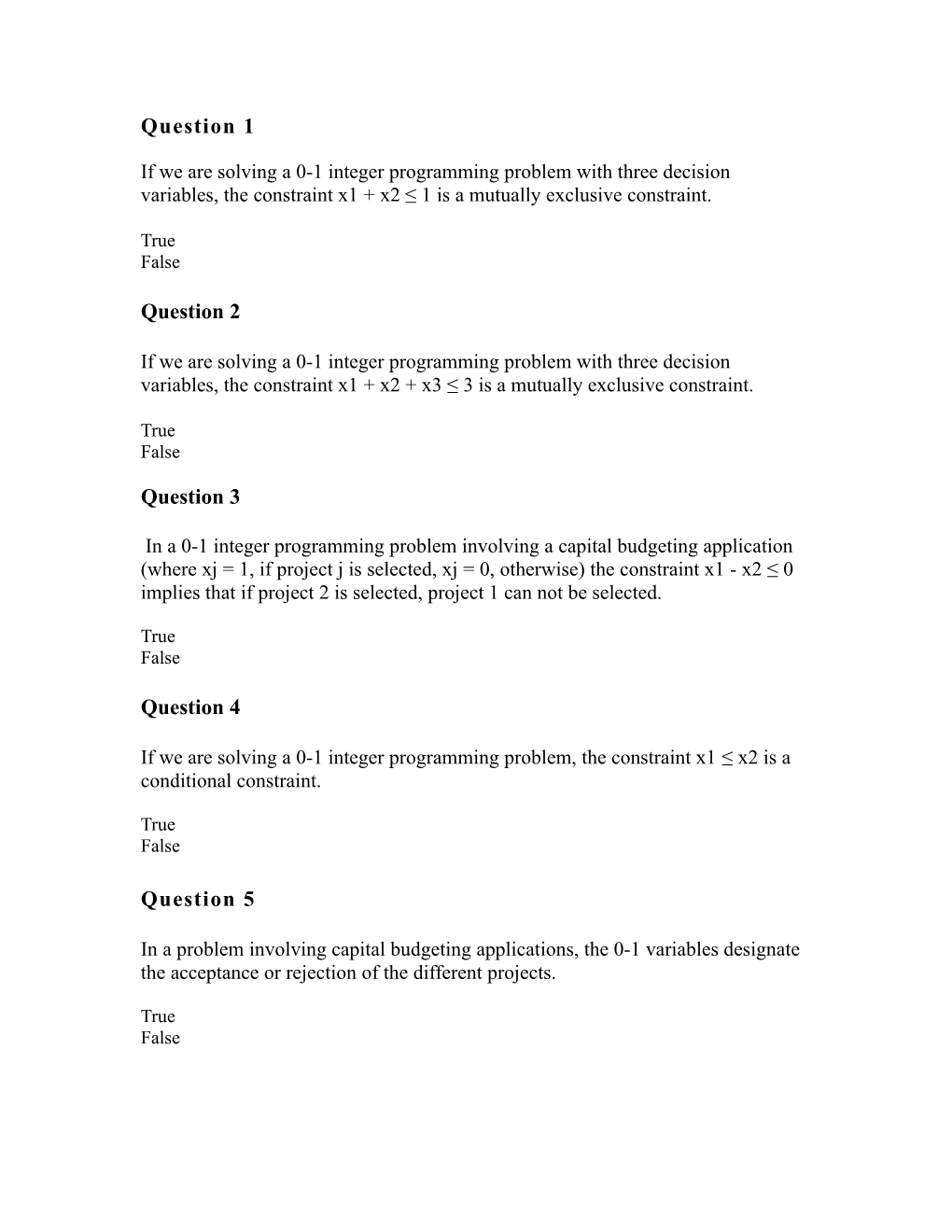# If We Are Solving a 0-1 Integer Programming Problem, the Constraint X1 X2is a ConditionalQuestion 1

If we are solving a 0-1 integer programming problem with three decision variables, the constraint x1+ x2≤ 1 is a mutually exclusive constraint.

True

False

Question 2

If we are solving a 0-1 integer programming problem with three decision variables, the constraint x1+ x2+ x3≤ 3 is a mutually exclusive constraint.

True

False

Question 3

In a 0-1 integer programming problem involving a capital budgeting application (where xj= 1, if project j is selected, xj= 0, otherwise) the constraint x1- x2≤ 0 implies that if project 2 is selected, project 1 can not be selected.

True

False

Question 4

If we are solving a 0-1 integer programming problem, the constraint x1≤ x2is a conditional constraint.

True

False

Question 5

In a problem involving capital budgeting applications, the 0-1 variables designate the acceptance or rejection of the different projects.

True

False

Question 6

A conditional constraint specifies the conditions under which variables are integers or real variables.

True

False

Question 7

If we are solving a 0-1 integer programming problem, the constraint x1+ x2= 1 is a ______constraint.

*multiple choice

*mutually exclusive

*conditional

*corequisite

Question 8

Binary variables are:

.0 or 1 only
.any integer value
.any continuous value
.any negative integer value

2 points

Question 9

In a 0-1 integer programming model, if the constraint x1-x2= 0, it means when project 1 is selected, project 2 ______be selected.

.can also
.can sometimes
.can never
.must also

Question 10

In a ______integer model, some solution values for decision variables are integers and others can be non-integer.

.total
.0 - 1
.mixed
.all of the above

Question 11

If we are solving a 0-1 integer programming problem, the constraint x1≤ x2is a ______constraint.

.multiple choice
.mutually exclusive
.conditional
.corequisite

Question 12

If the solution values of a linear program are rounded in order to obtain an integer solution, the solution is:

.always optimal and feasible
.sometimes optimal and feasible
.always optimal but not necessarily feasible
.never optimal and feasible

Question 13

Assume that we are using 0-1 integer programming model to solve a capital budgeting problem and xj= 1 if project j is selected and xj= 0, otherwise.

The constraint (x1 + x2 + x3 + x4 ≤ 2) means that ______out of the 4 projects must be selected.

.exactly 2
.at least 2
.at most 2
.none of the above

Question 14

The Wiethoff Company has a contract to produce 10000 garden hoses for a customer. Wiethoff has 4 different machines that can produce this kind of hose. Because these machines are from different manufacturers and use differing technologies, their specifications are not the same.

Write a constraint to ensure that if machine 4 is used, machine 1 will not be used.

.Y1+ Y4 ≤0
.Y1+ Y4 = 0
.Y1+ Y4 ≤1
.Y1+ Y4 ≥ 0

Question 15

The Wiethoff Company has a contract to produce 10000 garden hoses for a customer. Wiethoff has 4 different machines that can produce this kind of hose. Because these machines are from different manufacturers and use differing technologies, their specifications are not the same.

Write the constraint that indicates they can purchase no more than 3 machines.

.Y1+ Y2 + Y3+ Y4 ≤3
.Y1+ Y2 + Y3+ Y4 =3
.Y1+ Y2 + Y3+ Y4 ≥3
.none of the above

Question 16

Max Z = 5x1+ 6x2

Subject to: 17x1+ 8x2≤ 136

3x1+ 4x2≤ 36

x1, x2≥ 0 and integer

What is the optimal solution?

.x1 = 6, x2 = 4, Z = 54
.x1 = 3, x2 = 6, Z = 51
.x1 = 2, x2 = 6, Z = 46
.x1 = 4, x2 = 6, Z = 56

Question 17

You have been asked to select at least 3 out of 7 possible sites for oil exploration. Designate each site as S1, S2, S3, S4, S5, S6, and S7. The restrictions are:

Restriction 1. Evaluating sites S1and S3will prevent you from exploring site S7.

Restriction 2. Evaluating sites S2 orS4 will prevent you from assessing site S5.

Restriction 3. Of all the sites, at least 3 should be assessed.

Assuming that Siis a binary variable, the constraint for the first restriction is

.S1+ S3+ S7≥ 1
.S1+ S3+ S7≤1
.S1+ S3+ S7= 2
.S1+ S3+ S7≤ 2

Question 18

In a 0-1 integer programming model, if the constraint x1-x2≤ 0, it means when project 2 is selected, project 1 ______be selected.

.must always
.can sometimes
.can never
.A and B

Question 19

Consider the following integer linear programming problem:

Max Z = 3x1+ 2x2

Subject to: 3x1+ 5x2 ≤ 30

5x1+ 2x2 ≤ 28

x1 ≤ 8

x1,x2 ≥ 0 and integer

What is the optimal solution? Write your answer in the form :( x1, x2, z). (For example, the expression (10, 20, 50) means that x1 = 10, x2 = 20, and z = 50).

Question 20

Max Z = 3x1+ 5x2

Subject to: 7x1+ 12x2≤ 136

3x1+ 5x2≤ 36

x1, x2≥ 0 and integer

Find the optimal solution. Write your answer in the form :( x1, x2, z). (For example, the expression (10, 20, 50) means that x1 = 10, x2 = 20, and z = 50).